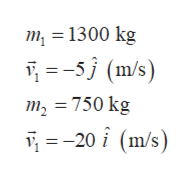Two cars collide at an icy intersection and stick together afterward. The first car has a mass of 1300 kg and was approaching at 5.00 m/s due south. The second car has a mass of 750 kg and was approaching at 20.0 m/s due west.(a) Calculate the final velocity of the cars. (Note that since both cars have an initial velocity, you cannot use the equations for conservation of momentum along the x-axis and y-axis; instead, you must look for other simplifying aspects..) Magnitude ....................m/s Direction  .....................Â° (counterclockwise from west is positive) (b) How much kinetic energy is lost in the collision? (This energy goes into deformation of the cars.) .........................J

Question

Two cars collide at an icy intersection and stick together afterward. The first car has a mass of 1300 kg and was approaching at 5.00 m/s due south. The second car has a mass of 750 kg and was approaching at 20.0 m/s due west.

(a) Calculate the final velocity of the cars. (Note that since both cars have an initial velocity, you cannot use the equations for conservation of momentum along the x-axis and y-axis; instead, you must look for other simplifying aspects..)
Magnitude
....................m/s
Direction
.....................Â° (counterclockwise from west is positive)

(b) How much kinetic energy is lost in the collision? (This energy goes into deformation of the cars.)
.........................J

Step 1
• Determine the final velocity of the cars after collision and their direction.
• Determine the loss in kinetic energy that occurred during collision.
Step 2

According to the information given in the question,help_outlineImage Transcriptionclosem 1300 kg -5j (m/s = 750 kg 20m/s) fullscreen
Step 3

Let car 1 of mass m1, and car 2 of mass m2, be moving with velocity v1 and v2, respectively.

Let vf be the final velocity of both the cars after colli...

Want to see the full answer?

See Solution

Want to see this answer and more?

Our solutions are written by experts, many with advanced degrees, and available 24/7

See Solution
Tagged in

Newtons Laws of Motion